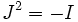# Almost Hermitian manifold

This article defines a differential manifold with the following additional structure -- the structure group is reduced to: unitary group

## Definition

An almost Hermitian manifold is the following data:

• A differential manifold$M$
• A Riemannian metric$g$ on$M$
• An almost complex structure viz a map$J: TM \to TM$ such that$J^2 = -I$ with the further property that$g(Jv,w) + g(v,Jw) = 0$

Note that this gives a reduction of the structure group to the unitary group over complex numbers.